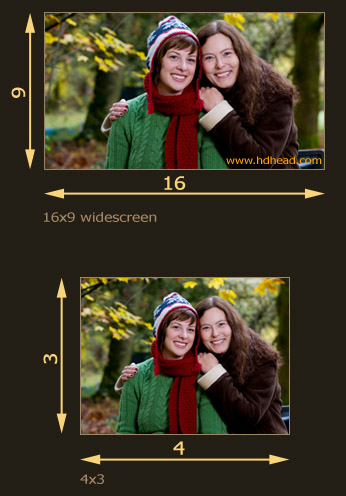# Aspect Ratios

1.78 for HD, 1.33 for SD.

Aspect ratio is the quotient of screen’s width and screen’s height. For example, a standard definition frame is 4 units wide and 3 units tall which is 4:3=1.33. Aspect ratio for SD is commonly expressed as either 1.33, 4×3 or 4:3.

The HD screen is wider. It is 16 units wide and 9 units tall which is: 16:9=1.78. You will see any of these: 1.78, 16×9 or 16:9. Sometimes you may run into 1.77 which is practically the same as 1.78.

The difference in aspect ratios between HD and SD requires you to specify the target aspect ratio when creating SD downconversions.Fig.1. High Definition and Standard Definition Aspect Ratios